#### You may also like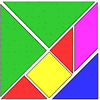### Tangrams

Can you make five differently sized squares from the interactive tangram pieces?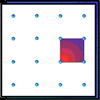### Geoboards

This practical challenge invites you to investigate the different squares you can make on a square geoboard or pegboard.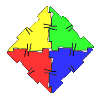### Polydron

This activity investigates how you might make squares and pentominoes from Polydron.

# Fitted

##### Age 7 to 11Challenge Level
We have had some good solutions for this challenge.

Here is what Ella from Neil Cummins school in the USA”¨ wrote:

I figured out the problem by first finding the area of all of the squares. Once I figured out the area of the squares, I added all of the areas together.  At first I got 956 for the area, but when Mr. Owens showed us what other students had done, the right answer for the area was shown on the screen.  It turned out that I had forgotten to add in the area of the 10 by 10 square (100).

I then figured out that you have to find all of the factors for the total area.  Mr. Owens showed us all of the factors for 1056, which is the total area.  He also mentioned that the sides of the rectangle could be bigger than 18  (which is the dimensions of the biggest square).  I tried dividing that by 18, but the answer turned out to be 58 r 12.  After I had listed them down, I thought that 32 x 33 would be the most likely of all.  I then drew a rectangle with those dimensions, and tried experimenting.  At first, I wasn't sure where to put the 18 square, but my friends Dakota and Hannah suggested that I put it in a corner.  So I started by putting the 18 square in the top left corner.  Since 18 + 18 is 36, I knew that 18 + 14 is 32.  Therefore, underneath the 18 square, I put the 14 square.  I put the 4 square right underneath the 18 square on the right side.  Then, since 4 + 10 is 14, I added the 10 square right underneath it.  I realised that the 9 square could fit beside the 10 square, so I put it there.  I made a mistake at first and made the dimensions of the 9 square 9 by 10.  Since it looked equal, I thought I was on to something.  After that, I tried to put the 15 square on top of the 10 square, but I knew it wouldn't work.  So then I tried the 7 square.  It fitted well, and there were 15 squares left on top of it.  There were 8 squares left over.  It was perfect for the 8.  But, I realised that the 8 was a square taller than the 7.  That was when I figured out that I had made a mistake.  So I corrected the 9 and 8 squares, and found the perfect spots for the 15 and 1 squares.  Then, I realised that it all fited!

Here's the result:

#####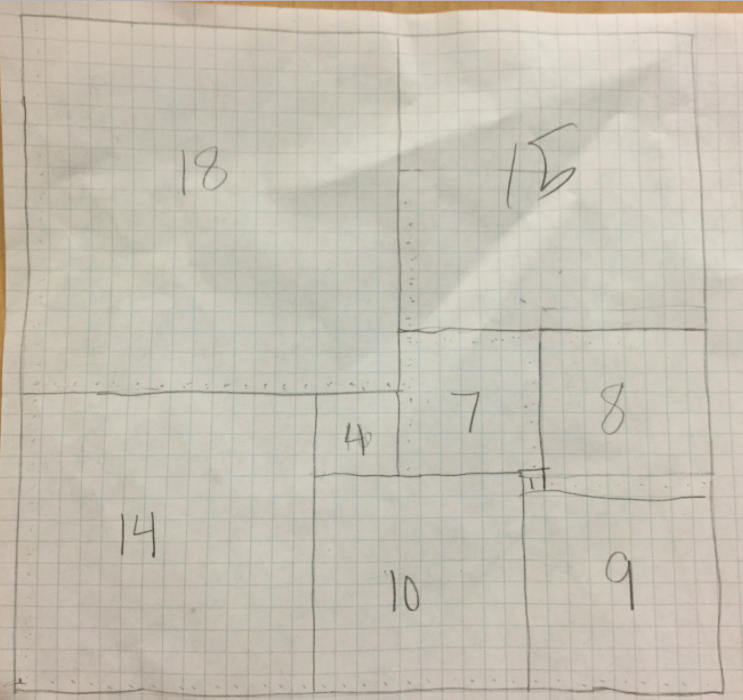Ella, that is written and explained very well. Well done!

Phoebe and Isobel from Greenacre School for Girls wrote the following:”¨

We worked out the area that the rectangle would be and then put some squares together to find possible lengths of the side of the rectangle. We knew we had to be able to divide the total area by the length of a side exactly, and checked that. When we put the 15x15 square next to the 18x18 square we got a length of 33cm and found this was a factor of the total area and that the other side had to be 32 cm, so we rearranged the squares until we had made that rectangle.

Here's the result: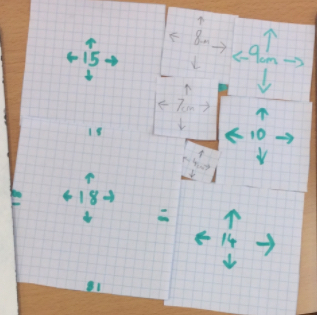Molly and Elizabeth from The Academy of Cuxton Schools”¨ sent in their thoughts:

We are proud to have solved this problem. We are exhilarated that we completed this task; it made us feel that any goal can be accomplished.

We completed this investigation by cutting out the squares and physically moving them into place. It took a lot of trial and improvement but we did it in the end.

We found one solution but if we kept trying, we believe we may find more. How many have you found?

Here's the result: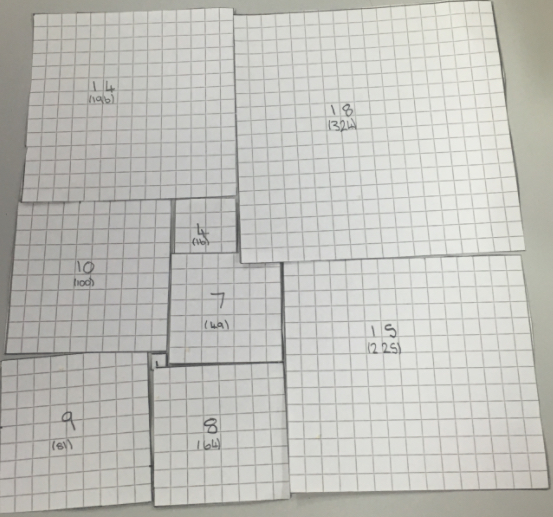Drew from Y6 Keston Primary sent in the following:-
I've worked out a solution for Fitted. I cut out the squares and managed to complete a  seamless square - actually a rectangle. Adding the dimensions gives 32cm x 33cm.

After I'd done this, I calculated the areas of all 9 squares and added them which totalled 1056cm2. I multiplied my shape's side lengths together i.e. 32x33 and it totalled 1056cm2!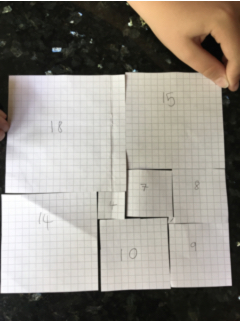Well done to everyone who sent in their results. It was good to read the different methods for getting a solution.